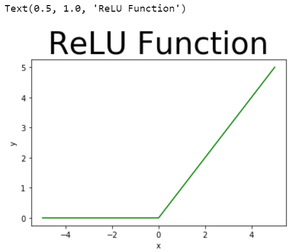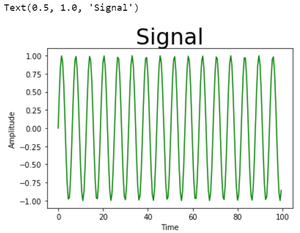# How to change the font size of the Title in a Matplotlib figure ?

• Last Updated : 03 Nov, 2021

In this article, we are going to discuss how to change the font size of the title in a figure using matplotlib module.

As we use matplotlib.pyplot.title() method to assign a title a plot, so in order to change the font size, we are going to use the fontsize argument of the pyplot.title() method in the matplotlib module.

Attention geek! Strengthen your foundations with the Python Programming Foundation Course and learn the basics.

To begin with, your interview preparations Enhance your Data Structures concepts with the Python DS Course. And to begin with your Machine Learning Journey, join the Machine Learning - Basic Level Course

Below is are some programs which depict how to change the font size of the title in a matplotlib figure:

Example 1:

## Python3

 `# importing module``import` `matplotlib.pyplot as plt` `# assigning x and y coordinates``x ``=` `[``-``5``, ``-``4``, ``-``3``, ``-``2``, ``-``1``, ``0``, ``1``, ``2``, ``3``, ``4``, ``5``]``y ``=` `[]` `for` `i ``in` `range``(``len``(x)):``    ``y.append(``max``(``0``, x[i]))` `# depicting the visualization``plt.plot(x, y, color``=``'green'``)``plt.xlabel(``'x'``)``plt.ylabel(``'y'``)` `# displaying the title``plt.title(``"ReLU Function"``,``          ``fontsize ``=` `40``)`

Output:Example 2:

## Python3

 `# importing modules``from` `matplotlib ``import` `pyplot as plt` `# assigning x and y coordinates``foodPreference ``=` `[``'Vegetarian'``, ``'Non Vegetarian'``,``                                ``'Vegan'``, ``'Eggitarian'``]` `consumers ``=` `[``30``, ``100``, ``10``, ``60``]` `# depicting the visualization``fig ``=` `plt.figure()``ax ``=` `fig.add_axes([``0``, ``0``, ``1``, ``1``])``ax.axis(``'equal'``)``ax.pie(consumers, labels ``=` `foodPreference,``       ``autopct``=``'%1.2f%%'``)` `# displaying the title``plt.title(``"Society Food Preference"``,``          ``fontsize ``=` `10``)`

Output:Example 3:

## Python3

 `# importing modules``from` `matplotlib ``import` `pyplot``import` `numpy` `# assigning time values of the signal``# initial time period, final time period``# and phase angle``signalTime ``=` `numpy.arange(``0``, ``100``, ``0.5``)` `# getting the amplitude of the signal``signalAmplitude ``=` `numpy.sin(signalTime)` `# depicting the visualization``pyplot.plot(signalTime, signalAmplitude,``            ``color ``=` `'green'``)` `pyplot.xlabel(``'Time'``)``pyplot.ylabel(``'Amplitude'``)` `# displaying the title``pyplot.title(``"Signal"``,``             ``fontsize ``=` `30``)`

Output:My Personal Notes arrow_drop_up